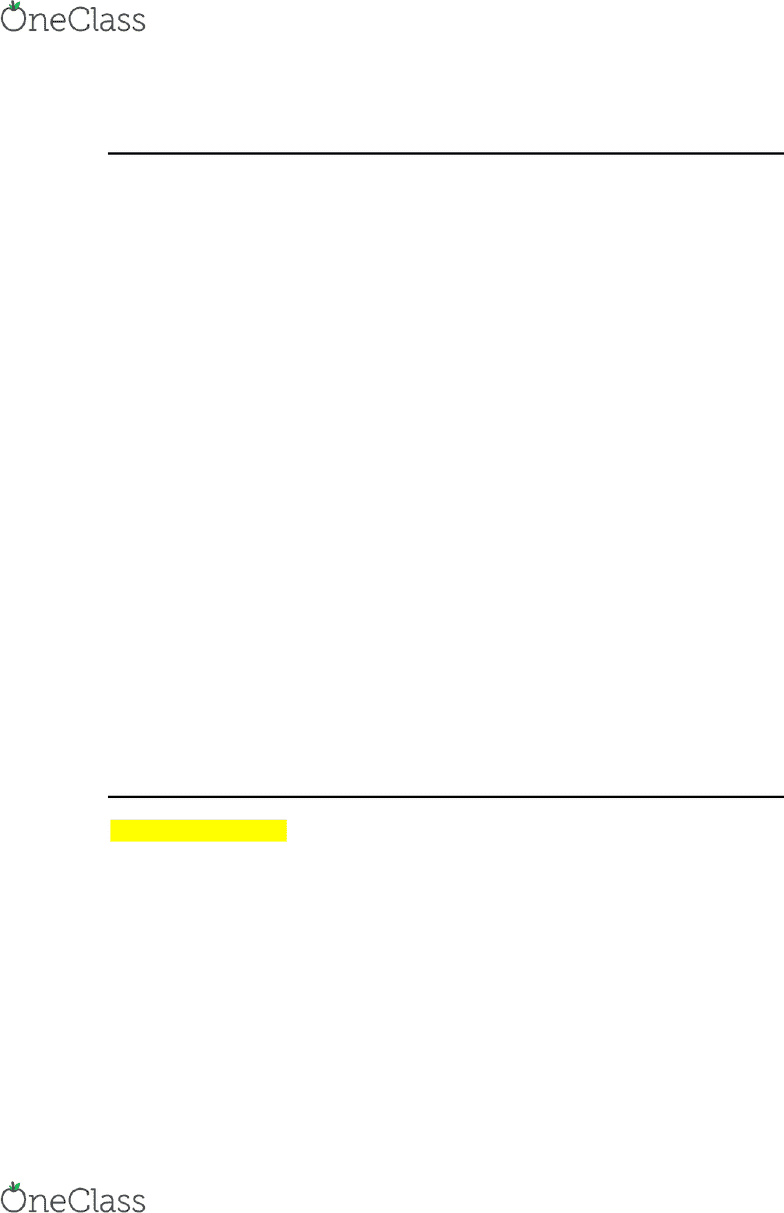# ACTG 2300 Lecture 18: Day 18 Notes

25 views4 pages
School
Department
Course

For unlimited access to Class Notes, a Class+ subscription is required.Day 18 3/5
Revenue Variance = Flexible Budget Revenues Actual Revenues
Spending Variance = Actual Cost Flexible Budget Cost
DM
o Price Variance
(Actual Price STD Price) * Total Actual Quantity
o Quantity Variance
(Total Quantity STD Quantity Allowed) * STD Price
DL
o Rate Variance
(Actual Rate STD Rate) * Total Actual Time
o Efficiency Variance
(Total Actual Time STD Time Allowed) * STD Rate
VOH
o Rate Variance
(Actual MOH Cost Rate STD MOH Cost Rate) * Total Actual
Cost Driver
STD MOH Cost Rate = Estimated MOH Cost Rate
o Efficiency Variance
(Total Actual Cost Driver STD Cost Driver Allowed) * STD
MOH Rate
Flexible Budget = Budgeted Cost * Actual Volume
Flexible Budget answers the question what would have the budget been if I
knew the actual volume?
***STD --- Allowed = standard --- allowed by the actual Volume
Exercise 10-2 (p. 451)
Spending Variance DL Cost = Actual Cost Flexible Budget Cost
Flexible Budget Cost
4,000 meals * (\$9.75 / meal * .25 DL hours) = \$9,750
The DL Cost that we would expect to have if we knew the actual V of 4,000
Actual Cost
\$9,600
Spending Variance DL Cost = \$9,750 - \$9,600 = \$150 favorable
Favorable because we planned for more and used less
find more resources at oneclass.com
find more resources at oneclass.com
Unlock document

This preview shows page 1 of the document.
Unlock all 4 pages and 3 million more documents.

Already have an account? Log in

# Get access

Grade+
\$10 USD/m
Billed \$120 USD annually
Homework Help
Class Notes
Textbook Notes
40 Verified Answers
Study Guides
1 Booster Class
Class+
\$8 USD/m
Billed \$96 USD annually
Homework Help
Class Notes
Textbook Notes
30 Verified Answers
Study Guides
1 Booster Class